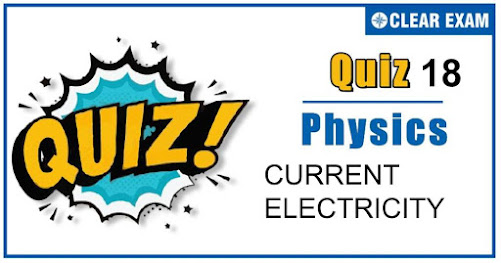## [LATEST]\$type=sticky\$show=home\$rm=0\$va=0\$count=4\$va=0

As per analysis for previous years, it has been observed that students preparing for NEET find Physics out of all the sections to be complex to handle and the majority of them are not able to comprehend the reason behind it. This problem arises especially because these aspirants appearing for the examination are more inclined to have a keen interest in Biology due to their medical background. Furthermore, sections such as Physics are dominantly based on theories, laws, numerical in comparison to a section of Biology which is more of fact-based, life sciences, and includes substantial explanations. By using the table given below, you easily and directly access to the topics and respective links of MCQs. Moreover, to make learning smooth and efficient, all the questions come with their supportive solutions to make utilization of time even more productive. Students will be covered for all their studies as the topics are available from basics to even the most advanced. .

Q1. It is easier to start a car engine on a hot day than on a cold day. This is because the internal resistance of the car battery?
•  Decreases with rise in temperature
•  Increases with rise in temperature
•  Decreases with a fall in temperature
•  Does not change with a change in temperature
Solution
Internal resistance ∝1/Temperature

Q2. In the circuit shown, the current though 8 ohm is same before and after connecting E. The value of E is?
•  12 V
•  6 V
•  4 V
•  2 V
Solution
Before connecting E, the circuit diagram is as shown in the figure.
The equivalent resistance of the given circuit is Req=6 Ω+8 Ω+10 Ω=24 Ω Current in the circuit, I=12V/24Ω=1/2 A Before connecting E, the current through 8 Ω is I=1/2 A After connecting E, the current through 8 Ω is also I=1/2 A ∴E=1/2 A×8Ω=4V

•  A
•  B
•  C
•  None of These
Solution
At point A the slope of the graph will be negative. Hence resistance is negative

Q4. A thin wire of resistance 4 Ω is bent to form a circle. The resistance across any diameter is?
•  4 Ω
•  2 Ω
•  1 Ω
•  8 Ω
Solution
Given that the resistance of the total wire is 4Ω.
Here, ACB(2Ω) and ADB (2Ω) are in parallel. So, the resistance across any diameter is 1/R=1/2+1/2=2/2=1 R=1Ω

Q5. The current flowing through a wire depends on time as I=3t2+2t+5. The charge flowing through the cross-section of the wire in time from t=0 to t=2 sec. is?
•  22 C
•  20 C
•  18 C
•  5 C
Solution

Q6. How much work is required to carry a 6 μC charge from the negative terminal to the positive terminal of a 9 V battery?
•  54×10-3 J
•  54×10-6 J
•  54×10-9 J
•  54×10-12 J
Solution
W=qV=6×10-6×9=54×10-6 J

Q7. The direction of current in an iron-copper thermocouple is?
•  From copper to iron at the hot junction
•  From iron to copper at the hot junction
•  From copper to iron at cold junction
•  No current will flow
Solution
From copper to iron at the hot junction

Q8. There resistances of 4 Ω each are connected as shown in figure. If the point D divides the resistance into two equal halves, the resistance between points A and D will be?
•  12 Ω
•  6 Ω
•  3 Ω
•  1/3 Ω
Solution
The equivalent circuit is given by
4Ω and 2Ω resistances are in series on both sides. ∴4Ω+2Ω=6Ω Then 6Ω and 6Ω resistances are in parallel on both sides 1/R=1/6+1/6=2/6=1/3

Q9. Four wires AB,BC,CD,DA of resistance 4 ohm each and a fifth wire BD of resistance 8 ohm are joined to form a rectangle ABCD of which BD is a diagonal. The effective resistance between the points A and B is?
•  24 ohm
•  16 ohm
•  4/3 ohm
•  8/3 ohm
Solution
Effective resistance between the points A and B is R=32/12=8/3 Ω

Q10. If the emf of a thermocouple, one junction of which is kept 0℃ is given by e=at+1/2 bt2, then the neutral temperature will be?
•  a/b
•  -a/b
•  a/2b
•  -1/ab
Solution
When temperature of the hot junction of a thermocouple rises, the thermo-emf increases and becomes maximum at a particular temperature. This temperature is called neutral temperature. Given, e=at+1/2 bt2 Differentiating with respect to t, we get de/dt=a+bt For maximum value of e, de/dt=0 ∴a+bt=0 ⇒t=-a/b## Want to know more

Please fill in the details below:

## Latest NEET Articles\$type=three\$c=3\$author=hide\$comment=hide\$rm=hide\$date=hide\$snippet=hide

Name

ltr
item
BEST NEET COACHING CENTER | BEST IIT JEE COACHING INSTITUTE | BEST NEET & IIT JEE COACHING: Current Electricity Quiz 18
Current Electricity Quiz 18